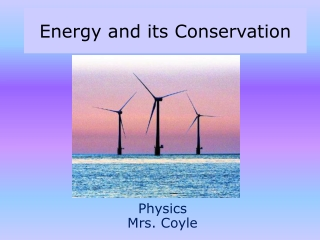DownloadDownload PresentationEnergy and its Conservation

# Energy and its Conservation

Download Presentation## Energy and its Conservation

- - - - - - - - - - - - - - - - - - - - - - - - - - - E N D - - - - - - - - - - - - - - - - - - - - - - - - - - -
##### Presentation Transcript

1. Energy and its Conservation PhysicsMrs. Coyle

2. Part I • Mechanical Energy • Potential • Kinetic • Work Energy Theorem

3. Energy and Work • Energy is the ability to do work. • Work is the energy transferred to or from a system by a force that acts on it.

5. Energy • Symbol: E • Scalar • Units: • J, Joule • cal, calorie • kcal, kilocalorie (Cal)

6. Mechanical Energy • Potential Energy • Kinetic Energy

7. Kinetic Energy, KE= 1 mv2 2Energy of Motion Coyle, Greece, 2005

8. Problem 1 A bird of mass 2kg is traveling at a speed of 6m/s. What is its kinetic energy? (A: 36J) If the speed of the bird doubles what happens to its kinetic energy?

9. Question • Can Kinetic Energy be Negative?

10. Work-Energy Theorem W=KE W=KEf-KEi The net work done on an object is equal to the change in its kinetic energy.

11. Problem 2 If Robin Hood applies a 15N force to pull his bow back by 25cm, with what speed will his 0.150kg arrow leave the bow?A: 7.1m/s

12. Problem 3 What is the work done by gravity as an 8 kg object falls from rest at 15m to 5m? What is the object’s speed at 5m? A: 800J, 14m/s

13. Work Done by the Gravitational Force System is an object and the earth. As an object falls: W= mgh As an object is thrown up: W= -mgh

14. Potential Energy, PE=mgh • Energy of position • Stored energy • Symbol: U or PE • Unit: Joule • Compared to a Reference Point (base level)

15. Problem 4 A flower pot of mass 5kg is located 15m above the ground. A)What is its potential energy with respect to the ground? B) What work was done to raise it to its position? A: 750J

16. Part II • Conservation of Energy

17. Conservation of Energy • Energy can neither be created nor destroyed. It can only change from form to form. • Mechanical Energy, PE and KE • Conservation of Mechanical Energy PE1 + KE1 = PE2 + KE2

18. What happens when friction is present? • When friction is present, the work done by the frictional force W=fd is transferred to heat energy. • Friction is a “non-conservative” force.

19. A Force is “Non-Conservative” if: • “the work it does on an object that moves between two points depends on the path taken.” • “the work it does on an object that moves through a round trip is non-zero.” • Example: friction, tension, normal force, propulsion forces.

20. A Force is “Conservative” if: • “ the work it does on an object that moves between two points depends only on the position of these two points and not on the path.” • “the work it does on an object that moves through a round trip is zero.” • Example: gravity, force of a spring.

21. Problem 5 A 3kg watermelon sits on a table 1.2m above the ground. • What is its PE at the top compared to the ground? b) What is its KE at the top? c) With what speed will it hit the ground?A: 36J, 0J, 4.9m/s

22. Problem 6 A penny is at the top of the Empire State Building 381m above the ground. It is then released. With what speed will it hit the ground? A: 87.3 m/s

23. Problem 7-Roller Coaster A roller coaster car started from point A at a height of 100m. What is its speed at point B? A: 44.7m/s

24. Problem 8 - Pendulum a) What is the potential energy of the bob at point A compared to the ground? b) What is the speed of the bob at the bottom?A: 2.3J, 1.1m/s A